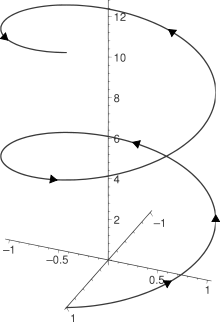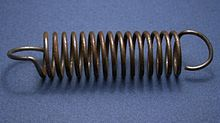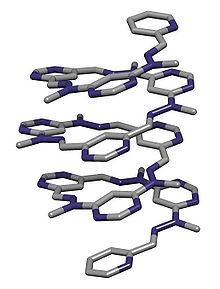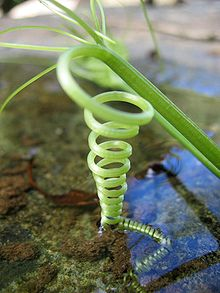# Helix

﻿
HelixThe helix (cos t, sin t, t) from t = 0 to 4π with arrowheads showing direction of increasing t.A helical coil springCrystal structure of a folded molecular helix reported by Lehn and coworkers in Helv. Chim. Acta., 2003, 86, 1598-1624.A natural left-handed helix, made by a climber plant.

A helix (pl: helixes or helices) is a type of smooth space curve, i.e. a curve in three-dimensional space. It has the property that the tangent line at any point makes a constant angle with a fixed line called the axis. Examples of helixes are coil springs and the handrails of spiral staircases. A "filled-in" helix – for example, a spiral ramp – is called a helicoid. Helices are important in biology, as the DNA molecule is formed as two intertwined helices, and many proteins have helical substructures, known as alpha helices. The word helix comes from the Greek word ἕλιξ, "twisted, curved".

## Types

Helices can be either right-handed or left-handed. With the line of sight along the helix's axis, if a clockwise screwing motion moves the helix away from the observer, then it is called a right-handed helix; if towards the observer then it is a left-handed helix. Handedness (or chirality) is a property of the helix, not of the perspective: a right-handed helix cannot be turned or flipped to look like a left-handed one unless it is viewed in a mirror, and vice versa.

Most hardware screw threads are right-handed helices. The alpha helix in biology as well as the A and B forms of DNA are also right-handed helices. The Z form of DNA is left-handed.

The pitch of a helix is the width of one complete helix turn, measured parallel to the axis of the helix.

A double helix consists of two (typically congruent) helices with the same axis, differing by a translation along the axis.

A conic helix may be defined as a spiral on a conic surface, with the distance to the apex an exponential function of the angle indicating direction from the axis. An example is the Corkscrew roller coaster at Cedar Point amusement park.

A circular helix, (i.e. one with constant radius) has constant band curvature and constant torsion.

A curve is called a general helix or cylindrical helix if its tangent makes a constant angle with a fixed line in space. A curve is a general helix if and only if the ratio of curvature to torsion is constant.

## Mathematical description

In mathematics, a helix is a curve in 3-dimensional space. The following parametrisation in Cartesian coordinates defines a helix:$x(t) = \cos(t),\,$$y(t) = \sin(t),\,$$z(t) = t.\,$

As the parameter t increases, the point (x(t),y(t),z(t)) traces a right-handed helix of pitch 2π and radius 1 about the z-axis, in a right-handed coordinate system.

In cylindrical coordinates (r, θ, h), the same helix is parametrised by:$r(t) = 1,\,$$\theta(t) = t,\,$$h(t) = t.\,$

A circular helix of radius a and pitch 2πb is described by the following parametrisation:$x(t) = a\cos(t),\,$$y(t) = a\sin(t),\,$$z(t) = bt.\,$

Another way of mathematically constructing a helix is to plot a complex valued exponential function (exi) taking imaginary arguments (see Euler's formula).[vague]

Except for rotations, translations, and changes of scale, all right-handed helices are equivalent to the helix defined above. The equivalent left-handed helix can be constructed in a number of ways, the simplest being to negate any one of the x, y or z components.

### Arc length, curvature and torsion

The length of a circular helix of radius a and pitch 2πb expressed in rectangular coordinates as$t\mapsto (a\cos t, a\sin t, bt), t\in [0,T]$

equals$T\cdot \sqrt{a^2+b^2}$, its curvature is$\frac{|a|}{a^2+b^2}$ and its torsion is$\frac{b}{a^2+b^2}.$

## Examples

In music, pitch space is often modeled with helices or double helices, most often extending out of a circle such as the circle of fifths, so as to represent octave equivalency.

Wikimedia Foundation. 2010.

Synonyms:

### Look at other dictionaries:

• hélix — hélix …   Dictionnaire des rimes

• Helix — ? Helix …   Википедия

• hélix — [ eliks ] n. m. • 1690; gr. helix « spirale » 1 ♦ Anat. Ourlet du pavillon de l oreille, décrivant un demi cercle en partant de la conque jusqu à la partie supérieure du lobule. 2 ♦ Zool. Escargot. ● hélix nom masculin (latin scientifique helix,… …   Encyclopédie Universelle

• Helix SF — is a quarterly American speculative fiction online magazine edited by William Sanders and Lawrence Watt Evans. The poetry editor is Bud Webster.Sanders began the magazine as a place where writers could publish things that none of the regular… …   Wikipedia

• helix — HÉLIX s.n. (anat.) Margine a pavilionului urechii. [< fr. hélix, cf. gr. helix]. Trimis de LauraGellner, 20.04.2005. Sursa: DN ﻿ HÉLIX s. n. margine a pavilionului urechii. (< fr. hélix) …   Dicționar Român

• hélix — m. anat. Repliegue semicircular en el borde del pabellón de la oreja. Medical Dictionary. 2011. hélix repliegue semicircular que forma el …   Diccionario médico

• helix — helix; helix·in; helix·om·e·ter; su·per·helix; …   English syllables

• Helix — He lix, n.; pl. L. {Helices}, E. {Helixes}. [L. helix, Gr. ?, ?, fr. ? to turn round; cf. L. volvere, and E. volute, voluble.] 1. (Geom.) A nonplane curve whose tangents are all equally inclined to a given plane. The common helix is the curve… …   The Collaborative International Dictionary of English

• Helix — Helix, OR U.S. city in Oregon Population (2000): 183 Housing Units (2000): 68 Land area (2000): 0.114338 sq. miles (0.296135 sq. km) Water area (2000): 0.000000 sq. miles (0.000000 sq. km) Total area (2000): 0.114338 sq. miles (0.296135 sq. km)… …   StarDict's U.S. Gazetteer Places

• Helix, OR — U.S. city in Oregon Population (2000): 183 Housing Units (2000): 68 Land area (2000): 0.114338 sq. miles (0.296135 sq. km) Water area (2000): 0.000000 sq. miles (0.000000 sq. km) Total area (2000): 0.114338 sq. miles (0.296135 sq. km) FIPS code:… …   StarDict's U.S. Gazetteer Places

• helix — a spiral thing, 1560s, from L. helix spiral, from Gk. helix (gen. helikos), related to eilein to turn, twist, roll, from PIE *wel ik , from root *wel to turn, revolve (see VULVA (Cf. vulva)) …   Etymology dictionary